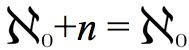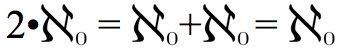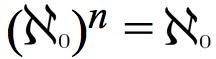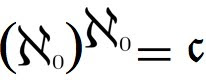Thursday, February 2, 2012

Infinity: Operations on Cardinals

Before reading this post, make sure you have read the first three parts of the Infinity Series, the first of which is found here. For all posts, see the Infinity Series Portal.

Having found the mathematical relationship between aleph-zero and the cardinality of the continuum, one wonders if it is possible to perform other operations with infinity cardinals, and whether these equations create any numbers not yet discussed. To start, take a simple addition from cardinal arithmetic:
(1)

What exactly does this equation mean? We are asked to "add" two quantities, one of which is an infinite cardinal, and one is a simple number. That it is possible to evaluate the sum (1) follows from the fact that aleph-zero and 1 are both cardinal numbers. Since they are of the same number system, they are "compatible" in a way, and can be combined by the use of sets. We have already proved (1) in the first post of the series, but let us recap. It has been discussed that adding the element 0 to the set of natural numbers does not change its cardinality, since the function y=x-1 from one set to the other is a bijection. In set notation, with vertical lines representing cardinality: |{0}|+|{1,2,3...}|=|{0,1,2,3...}| Note that if one replaces the values in this equation with the actual cardinalities, one obtains the equation (1) above! The equation in simple sets that has the same meaning as a cardinal equation will henceforth be known as the Corresponding Set Equality (CSE).

The generalization of (1) for any natural number n is(2)

This can also be transformed into a CSE, since any subset of integers also has cardinality aleph-zero. The the set {-n+1,-n+2,...-2,-1,0} clearly has n elements, and can be added to the natural numbers to form the CSE, namely: |{-n+1,-n+2,...-2,-1,0}|+|{1,2,3...}|=|{-n+1,-n+2,...-2,-1,0,1,2,3...}|. By evaluating the cardinalities on both sides, one obtains equation (2). It is easy to continue on to other arithmetic operations, such as multiplication:(3)

This particular equation also has a CSE. First consider the set of integers. It is clear that it can be split into two components, both of which have a cardinality of aleph-zero, namely the whole numbers: {0,1,2,3...} and the negative integers: {...-3,-2,-1} However, when these two sets are combined, the set of integers results, which we already know has cardinality aleph-zero as well. The CSE here is |{0,1,2,3...}|+|{-1,-2,-3...}|=|{...-3,-2,-1,0,1,2,3...}|. All three of the cardinalities are evaluated as aleph-zero, and (3) results. Now we shall prove the general multiplication result(4)

for any natural number n. We have already determined that the rational numbers, and any infinite subset of them have cardinality aleph-zero. Consider the set {a/n,1+(a/n),2+(a/n),...}. This is simply the set of natural numbers with the rational number a/n added to each term. For a constant n, one could consider creating a set for each integral value of a from 0, to n-1, inclusive. This produces n sets of cardinality aleph-zero.

An example will make this more clear. Consider the case with n=3. For a=0, the set produced is simply the whole numbers ({0/3,1+(0/3),2+(0/3)...}={0,1,2...}) For a=1, the set is {1/3,1+(1/3),2+(1/3)...} and for a=2, the set is {2/3,1+(2/3),2+(2/3)...}, all of which have cardinality aleph-zero. The sum of these sets is {0,1/3,2/3,1,1+(1/3),1+(2/3),2...}, or the set of all multiples of 1/3, which also has the same cardinality. We have just proved (4) for n=3. The more general CSE for (4) is |{0/n,1+(0/n),2+(0/n)...}|+|{1/n,1+(1/n),2+(1/n)...}|+
|{2/n,1+(2/n),2+(2/n)...}|+...+|{(n-1)/n,1+((n-1)/n),2+((n-1)/n)...}|=
|{0,1/n,2/n...,1,1+(1/n),1+(2/n),...}|, the final set being the set of multiples of 1/n.

This equation is tedious, but it simply is the division of the set of multiples of 1/n into n parts, all of which have cardinality of aleph-zero. Since the set of multiples on the right hand side of the equation as an equivalent cardinality, this proves (4). Moving on, even the multiplication of two infinite quantities is possible.(5)

The CSE for this equation follows from the countability of the set of ordered pairs. The set of ordered pairs (x,y) with natural numbers x and y can be split into components by setting a value for x, for example 1, and letting y vary among the natural numbers. For each constant value of x, a set of cardinality aleph-zero is generated, and since there are aleph-zero choices for x, the above result (5) follows. In CSE form, |{(1,1),(1,2),(1,3)...}|+|{(2,1),(2,2),(2,3)...}|+...=[the cardinality of the set of ordered pairs]. Each quantity in the equality has value aleph-zero when evaluated, and since there are as many members on the left side, it follows that the cardinality of the natural numbers, when multiplied by itself, yields the same quantity. This result can too be generalized to any positive integer power:(6)

Since the case n=2 makes use of ordered pairs, it is natural to assume that higher powers will involve the corresponding ordered n-tuplet. This is correct. There are aleph-zero possibilities for each element of an integral ordered n-tuplet, and each choice of element contributes an aleph-zero to the product. The end result is aleph-zero to the nth power, but since it has already been said that the cardinalities of the sets of ordered n-tuplets for finite n are all aleph-zero, the equality (6) is a direct result.

Summarizing the above, no additions, multiplications, or nth powers, when applied to aleph-zero, change its value. However, it has already been shown that two taken to the power of aleph-zero produces a different infinite cardinal, namely the cardinality of the continuum. But what about a general natural number n taken to the same power, or even aleph-zero taken to the power of itself?(7)

Remarkably, we find that this quantity is equal to the cardinality of the continuum! This can be derived intuitively from the result (6). Since aleph-zero to the power of n is equal to the cardinality of the set of the ordered n-tuplets, one obtains (7) by letting n increase without bound to aleph-zero, at which point one obtains the cardinality of the set of infinite sequences, which has previously been shown to be greater than aleph-zero, and named the cardinality of the continuum. Any other n taken to the aleph-zero power is also equal to the cardinality of the continuum, as such quantities would clearly be greater than 2 to that power and less than the left side of (7). Since these both have the same value, those of the general case do as well. This is all summarized below.The next post explores uncountable sets, namely stating what other sets besides the real or complex numbers have a cardinality equal to, or even greater than, the cardinality of the continuum.

Sources: http://en.wikipedia.org/wiki/Power_set